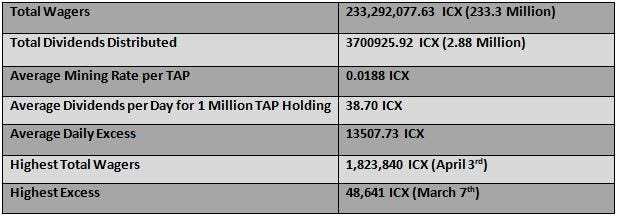# ICONbet Statistics for the period January 1st,2020 — Sep 30th, 2020

The below statistics have been compiled using the publicly available data and the data shared by the ICONbet community members.Average mining rate is calculated based on just the excess, if the developer fee is to be considered the average mining rate would be up to 20% more.

If you had 1 Million TAP by Dec 31st, 2019, you would have made 10603 ICX in the following 9 Months.

Following would be your ROI based on your cost of acquiring TAP.

If cost of 1 Million TAP = 12000 ICX.
ROI for 9 Months would be =(10603/12000)*100 =88.35%

If cost of 1 Million TAP = 15000 ICX.
ROI for 9 Months would be =(10603/15000)*100 =70.68%

If cost of 1 Million TAP = 20000 ICX.
ROI for 9 Months would be =(10603/20000)*100 =53.01%

If cost of 1 Million TAP = 25000 ICX.
ROI for 9 Months would be=(10603/25000)*100 =42.41%

If cost of 1 Million TAP = 30000 ICX.
ROI for 9 Months would be =(10603/30000)*100 =35.34%

If cost of 1 Million TAP = 35000 ICX.
ROI for 9 Months would be =(10603/35000)*100 =30.29%

If cost of 1 Million TAP = 40000 ICX.
ROI for 9 Months would be=(10603/40000)*100 =26.50%

If cost of 1 Million TAP = 45000 ICX.
ROI for 9 Months would be=(10603/45000)*100 =23.56%

If cost of 1 Million TAP = 50000 ICX.
ROI for 9 Months would be=(10603/50000)*100 =21.2%

Following are the Monthly Stats for the period January 1st,2020 — Sep 30th, 2020.